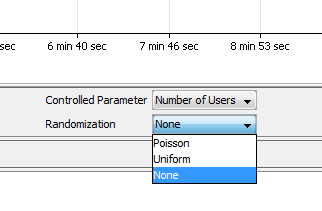# explanation of Uniform and Poisson Randomization in Loadtest

edited December 2016

This article will outline what the difference is between Poisson and Uniform Randomization in Loadtest. It will also show an example where a user might see an issue maintaining a specific Hits Per Minute when randomization is in use.

In LoadTest, there is an option below the "Controlled Parameter" dropdown to choose the randomization strategy.This setting is used to insert some degree of randomness into the controlled parameter you have selected.
There are two distinct flavors of randomization you can apply on the specific control parameter selected.

The LoadTest documentation for this option can be found here:
Loadtest Documentation > Customizing Loadtest Parameters > Customizing Test Suite Scenarios > Randomization

### For the Number of Users parameter,

The virtual user think time is randomized according to the following distributions:

Uniform: If the virtual user think time defined in the profile configuration is Tprof. then during load testing the actual virtual user think time will be a random value uniformly distributed in the interval from 0 to 2*Tprof. with the mean value of Tprof.

Poisson: If the virtual user think time defined in the profile configuration is Tprof. then during load testing the actual virtual user think time will be a random value with the bell shaped distribution and the mean value of Tprof.
None: No randomization is applied.

### For the Hits per Second parameter,

The inter-invocation time is randomized according to the following distributions:

Uniform: If the hit per second rate defined in the load test scenario is Nhps. then during load testing Load Test will generate a request sequence with a random
inter-invocation time (the time between subsequent requests) uniformly distributed
in the interval between 0 and 2* 1/Nhps. seconds and the mean value of 1/Nhps seconds.

Poisson: If the hit per second rate defined in the load test scenario is Nhps, then during load testing Load Test will generate a request sequence with a random
inter-invocation time (the time between subsequent requests) with the bell shaped
distribution and the mean value of 1/Nhps seconds.

Example
Lets say that a Loadtest is configured in the following way.
20 minutes
12 Hits Per Min
3 test steps

If you wanted to introduce some randomization into this scenario the math works out this way;

### Introducing Uniform Randomization

Inter-invocation time is the amount of time that expires between test runs
You would have 12 Hits/Min which is 0.2 Hits/sec
For 0 and 2* 1/Nhps we first need the inverse of 0.2 which is (1/n = 5)
0 and 5 So now its an inter-invocation time of 2.5 Hits/sec (the avg of 0 and 5)
3 tests * 24Hits/ Min (2.5 Hits/ sec / 60 secs) ~ 72 Hits Per Min

Notice how the Hits per minute is much different than 12? This is because the Think time between Test steps has been changed to chose a number between 0 and 5secs. This effectively overwrites the logic that Loadtest was going to use to determine how to execute the tests in order to achieve the 12 Hits per minute.